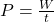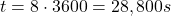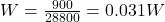## An electric motor does 900j of work for 8 hours. Calculate the power used​

Question

An electric motor does 900j of work for 8 hours. Calculate the power used​

in progress 0
5 months 2021-08-15T15:24:52+00:00 1 Answers 5 views 0

## Answers ( )

0.031 W

Explanation:

The power used is equal to the rate of work done:where

P is the power

W is the work done

t is the time taken to do the work W

In this problem, we have:

W = 900 J is the work done by the motor

t = 8 h is the time taken

We have to convert the time into SI units; keeping in mind that

1 hour = 3600 s

We haveAnd therefore, the power used is